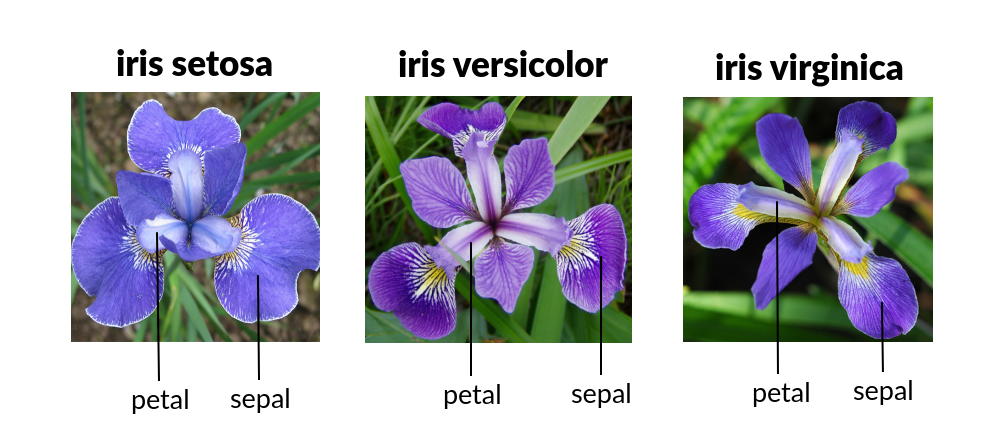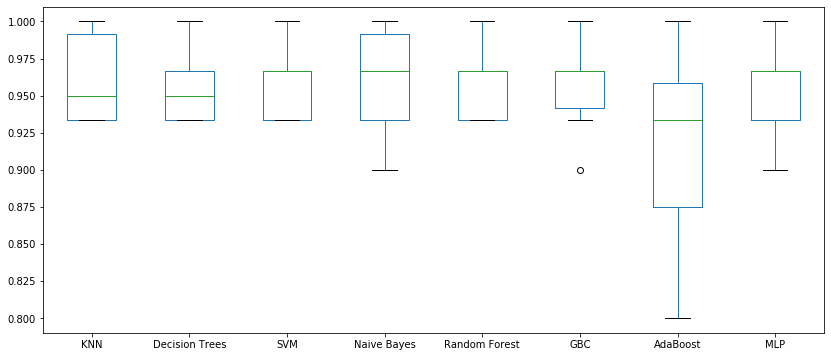# MLF - Spot-Checking Algorithms

Last updated: April 28th, 2020# Spot-checking algorithms¶

By having a correctly worked, clean and prepared dataset, we are ready to start the modeling phase to resolve a given problem.

As there is no algorithm that always offer optimal results, we'll test and evaluate a set of Machine Learning algorithms to try to solve our problem.

Doing this we will have (some more) security that our final model is optimal and we will have enough arguments to reject other alternatives.## Iris dataset¶

In this example we'll use the Iris dataset from UCI Machine Learning Repository, a really common and publicly available dataset for classification.

The dataset contains observations with the length and width of the petal and sepal of three iris species:

• sepal length in cm
• sepal width in cm
• petal length in cm
• petal width in cm

Given that dimensions of the flower, we will predict the species of the flower.

There are three Iris species:

• 0 = setosa
• 1 = versicolor
• 2 = virginicaIt's already built into the scikit learn package, we can just do load_iris().

Read more in scikit-learn docs.

In :
import numpy as np
import pandas as pd
%matplotlib inline

from sklearn import datasets
from sklearn.model_selection import train_test_split

In :
iris = datasets.load_iris()

In :
X = iris.data
y = iris.target

In :
X[:10]

Out:
array([[5.1, 3.5, 1.4, 0.2],
[4.9, 3. , 1.4, 0.2],
[4.7, 3.2, 1.3, 0.2],
[4.6, 3.1, 1.5, 0.2],
[5. , 3.6, 1.4, 0.2],
[5.4, 3.9, 1.7, 0.4],
[4.6, 3.4, 1.4, 0.3],
[5. , 3.4, 1.5, 0.2],
[4.4, 2.9, 1.4, 0.2],
[4.9, 3.1, 1.5, 0.1]])
In :
y[:10]

Out:
array([0, 0, 0, 0, 0, 0, 0, 0, 0, 0])

### Standardize data¶

For many machine learning algorithms, it is important to scale the data. Let's do that now using sklearn.

In :
from sklearn.preprocessing import StandardScaler

scaler = StandardScaler()

X = scaler.fit_transform(X)

In :
print(X.shape)

X[:10]

(150, 4)

Out:
array([[-0.90068117,  1.01900435, -1.34022653, -1.3154443 ],
[-1.14301691, -0.13197948, -1.34022653, -1.3154443 ],
[-1.38535265,  0.32841405, -1.39706395, -1.3154443 ],
[-1.50652052,  0.09821729, -1.2833891 , -1.3154443 ],
[-1.02184904,  1.24920112, -1.34022653, -1.3154443 ],
[-0.53717756,  1.93979142, -1.16971425, -1.05217993],
[-1.50652052,  0.78880759, -1.34022653, -1.18381211],
[-1.02184904,  0.78880759, -1.2833891 , -1.3154443 ],
[-1.74885626, -0.36217625, -1.34022653, -1.3154443 ],
[-1.14301691,  0.09821729, -1.2833891 , -1.44707648]])

Now that we've split the data, we can start "classifying" it.## Spot-check algorithms¶

We cannot know beforehand what algorithms will perform well on a given predictive modeling problem.

Spot-checking is an approach to this problem.

It involves rapidly testing a large suite of diverse machine learning algorithms on a problem in order to quickly discover what algorithms might work and where to focus attention.

• It is fast, it by-passes the days or weeks of preparation and analysis and playing with algorithms that may not ever lead to a result.
• It is objective, allowing you to discover what might work well for a problem rather than going with what you used last time.
• It gets results, you will actually fit models, make predictions and know if your problem can be predicted and what baseline skill may look like.

Scikit learn has a nice API that will allow you to swap different models in and out. We'll analyze a few and evaluate them by using a cross-validation approach with 10 stratified shuffle splits:

In :
from sklearn.model_selection import StratifiedShuffleSplit, cross_val_score

In :
def get_cv_scores(model):
return cross_val_score(model, X, y,
cv=StratifiedShuffleSplit(n_splits=10,
test_size=0.2,
random_state=10))

In :
results_df = pd.DataFrame()## K Nearest Neighbors¶

In :
from sklearn.neighbors import KNeighborsClassifier

model = KNeighborsClassifier()

In :
scores = get_cv_scores(model)

scores

Out:
array([1.        , 0.96666667, 0.96666667, 1.        , 0.93333333,
1.        , 0.93333333, 0.93333333, 0.93333333, 0.93333333])
In :
# print and store results

results_df['KNN'] = scores

print("Accuracy: %0.2f (+/- %0.2f)" % (scores.mean(), scores.std() * 2))

Accuracy: 0.96 (+/- 0.06)## Decision Trees¶

In :
from sklearn.tree import DecisionTreeClassifier

model = DecisionTreeClassifier(random_state=10)

In :
scores = get_cv_scores(model)

scores

Out:
array([1.        , 0.96666667, 0.93333333, 0.96666667, 0.93333333,
1.        , 0.93333333, 0.93333333, 0.93333333, 0.96666667])
In :
# print and store results

results_df['Decision Trees'] = scores

print("Accuracy: %0.2f (+/- %0.2f)" % (scores.mean(), scores.std() * 2))

Accuracy: 0.96 (+/- 0.05)## Support Vector Machines¶

In :
from sklearn import svm

model = svm.SVC(gamma='auto',
random_state=10)

In :
scores = get_cv_scores(model)

scores

Out:
array([1.        , 0.96666667, 0.96666667, 0.96666667, 0.93333333,
0.96666667, 0.93333333, 0.93333333, 0.96666667, 0.93333333])
In :
# print and store results

results_df['SVM'] = scores

print("Accuracy: %0.2f (+/- %0.2f)" % (scores.mean(), scores.std() * 2))

Accuracy: 0.96 (+/- 0.04)## Naive Bayes Classifier¶

In :
from sklearn.naive_bayes import GaussianNB

model = GaussianNB()

In :
scores = get_cv_scores(model)

scores

Out:
array([1.        , 1.        , 0.96666667, 0.96666667, 0.93333333,
1.        , 0.93333333, 0.9       , 0.93333333, 0.96666667])
In :
# print and store results

results_df['Naive Bayes'] = scores

print("Accuracy: %0.2f (+/- %0.2f)" % (scores.mean(), scores.std() * 2))

Accuracy: 0.96 (+/- 0.07)## Random Forest¶

In :
from sklearn.ensemble import RandomForestClassifier

model = RandomForestClassifier(n_estimators=100)

In :
scores = get_cv_scores(model)

scores

Out:
array([1.        , 0.96666667, 0.96666667, 0.96666667, 0.93333333,
1.        , 0.93333333, 0.93333333, 0.93333333, 0.96666667])
In :
# print and store results

results_df['Random Forest'] = scores

print("Accuracy: %0.2f (+/- %0.2f)" % (scores.mean(), scores.std() * 2))

Accuracy: 0.96 (+/- 0.05)## Gradient Boost Classifier¶

In :
from sklearn.ensemble import GradientBoostingClassifier

model = GradientBoostingClassifier(random_state=10)

In :
scores = get_cv_scores(model)

scores

Out:
array([1.        , 0.96666667, 0.96666667, 0.96666667, 0.93333333,
1.        , 0.93333333, 0.96666667, 0.9       , 0.96666667])
In :
# print and store results

results_df['GBC'] = scores

print("Accuracy: %0.2f (+/- %0.2f)" % (scores.mean(), scores.std() * 2))

Accuracy: 0.96 (+/- 0.06)## AdaBoost Classifier (Adaptive Boosting)¶

In :
from sklearn.ensemble import AdaBoostClassifier

model = AdaBoostClassifier(random_state=10)

In :
scores = get_cv_scores(model)

scores

Out:
array([1.        , 0.93333333, 0.96666667, 0.86666667, 0.93333333,
1.        , 0.86666667, 0.9       , 0.8       , 0.93333333])
In :
# print and store results

results_df['AdaBoost'] = scores

print("Accuracy: %0.2f (+/- %0.2f)" % (scores.mean(), scores.std() * 2))

Accuracy: 0.92 (+/- 0.12)## Neural Networks: Multi-layer Perceptron classifier¶

In :
from sklearn.neural_network import MLPClassifier

model = MLPClassifier(hidden_layer_sizes=(64,32),
max_iter=1000,
random_state=10)

In :
scores = get_cv_scores(model)

scores

Out:
array([1.        , 0.96666667, 0.96666667, 0.96666667, 0.93333333,
1.        , 0.96666667, 0.93333333, 0.93333333, 0.9       ])
In :
# print and store results

results_df['MLP'] = scores

print("Accuracy: %0.2f (+/- %0.2f)" % (scores.mean(), scores.std() * 2))

Accuracy: 0.96 (+/- 0.06)## Comparison of algorithms¶

In :
results_df

Out:
KNN Decision Trees SVM Naive Bayes Random Forest GBC AdaBoost MLP
0 1.000000 1.000000 1.000000 1.000000 1.000000 1.000000 1.000000 1.000000
1 0.966667 0.966667 0.966667 1.000000 0.966667 0.966667 0.933333 0.966667
2 0.966667 0.933333 0.966667 0.966667 0.966667 0.966667 0.966667 0.966667
3 1.000000 0.966667 0.966667 0.966667 0.966667 0.966667 0.866667 0.966667
4 0.933333 0.933333 0.933333 0.933333 0.933333 0.933333 0.933333 0.933333
5 1.000000 1.000000 0.966667 1.000000 1.000000 1.000000 1.000000 1.000000
6 0.933333 0.933333 0.933333 0.933333 0.933333 0.933333 0.866667 0.966667
7 0.933333 0.933333 0.933333 0.900000 0.933333 0.966667 0.900000 0.933333
8 0.933333 0.933333 0.966667 0.933333 0.933333 0.900000 0.800000 0.933333
9 0.933333 0.966667 0.933333 0.966667 0.966667 0.966667 0.933333 0.900000
In :
results_df.boxplot(figsize=(14,6), grid=False)

Out:
<matplotlib.axes._subplots.AxesSubplot at 0x7f971c654670>## Summarize¶

Now, we will analyze other ways to evaluate several models.

First, we need to prepare the models previously described

In :
# prepare models
models = []
models.append(('KNN', KNeighborsClassifier()))
models.append(('CART', DecisionTreeClassifier(random_state=10)))
models.append(('NB', GaussianNB()))
models.append(('SVM', svm.SVC(gamma='auto',
random_state=10)))
models.append(('GBC', GradientBoostingClassifier(random_state=10)))
models.append(('RF', RandomForestClassifier(n_estimators=100)))
models.append(('ADA',  AdaBoostClassifier(random_state=10)))
models.append(('MLP',  MLPClassifier(hidden_layer_sizes=(64,32),
max_iter=1000,
random_state=10)))


Finally, we will evaluate the accuracy of these models.

We will make a list with each algorithm short name, the mean accuracy and the standard deviation accuracy.

In :
# evaluate each model in turn
from sklearn.metrics import accuracy_score
results = []
names = []
scoring = 'accuracy'
for name, model in models:
scores = get_cv_scores(model)
names.append(name)
print(name, "Accuracy: %0.3f (+/- %0.3f)" % (scores.mean(), scores.std() *2))

KNN Accuracy: 0.960 (+/- 0.058)
CART Accuracy: 0.957 (+/- 0.052)
NB Accuracy: 0.960 (+/- 0.065)
SVM Accuracy: 0.957 (+/- 0.043)
GBC Accuracy: 0.960 (+/- 0.058)
RF Accuracy: 0.953 (+/- 0.061)
ADA Accuracy: 0.920 (+/- 0.120)
MLP Accuracy: 0.957 (+/- 0.060)